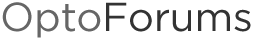# Two's complement calculation for LRC (alternate checksum method)

A question from a customer on 2s complement calculation, as an alternate to Checksum…

Note: PAC Control has a variety of commands in the “String Commands” category like “Generate Checksum on String” and “Generate Forward/Reverse CCITT/CRC-16” … but if you need something a little different, like LRC, you can always roll your own.

As the help text for “[B]Generate Checksum on String” [/B]says:

• To calculate the LRC of a string, take the two’s complement of the checksum:
[INDENT=2][B]1. [/B]Generate checksum on the string. [B]2. [/B]Subtract the checksum from 255. This is the one’s complement of the checksum. [B]3. [/B]Add one to the result. This is the two’s complement of the checksum. Example: For a string containing only the capital letter “A”, the checksum is 65. To calculate the LRC, subtract the checksum (65) from 255, which equals 190. Add one to this result, resulting in an LRC of 191. [/INDENT]

Here’s some OptoScript code to show what that example might look like in a strategy:

``````
sTxRx = "A";

nChecksum =  GenerateChecksumOnString(/*Start Value*/0, /*On String*/sTxRx);
nLRC = (255 - nChecksum) + 1; // calculate single-byte two's complement of checksum

// append that byte to the end of the string for transmitting:
sTxRx = sTxRx + chr(nLRC);

``````

Generally, in OptoScript a 1s complement is just the bitnot of the thing to take the complement of, like we do w/setting IP addresses [U][B]in this post[/B][/U] specifically this line: ntMemMapData = bitnot nIPAddress; // 1s Complement

And a 2s complement is just the 1s complement + 1. (In that help text above, they’re talking about one byte so a bitnot is the same as “subtract from 255.") Since our 32-bit (4 byte) integers are signed, you have to worry about things like sign extension on the rest of those 32-bits. So we could’ve also done, as an alternate to the last couple lines above:

``````
// calculate the 2s complement of the checksum, and append that to the string itself
sTxRx = sTxRx + chr( 0xFF bitand ( (bitnot nChecksum) + 1 ) );
``````

Anyway, happy coding and complementing!
-OptoMary

More two’s complement fun.

Will this loop ever exit?

``````
while(AbsoluteValue(i)>=0)
DecrementVariable(i);
wend

``````

Haven’t actually tried it (would that be cheating, Philip?) but I suspect this is a little bit of a trick question.
If i is an integer, I’d expect the loop to exit at i=0. If it’s a float, you might never get to zero. Is that the answer you got?

Oops, I messed up my comparison, it should be >= 0. I did test it, but I didn’t start with positive numbers. I’ll edit the post to fix it, and i is an integer.